## how many roots does this has? x^2+(2√5x)+2x=-10​ find Discriminant

Question

how many roots does this has?
x^2+(2√5x)+2x=-10​
find Discriminant

in progress 0
1 month 2021-08-31T12:21:35+00:00 1 Answers 0 views 0

1. Given:

The equation is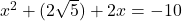To find:

The number of roots and discriminant of the given equation.

Solution:

We have,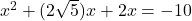The highest degree of given equation is 2. So, the number of roots is also 2.

It can be written as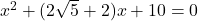Here,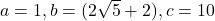.

Discriminant of the given equation is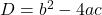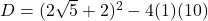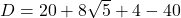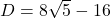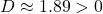Since discriminant is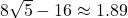, which is greater than 0, therefore, the given equation has two distinct real roots.